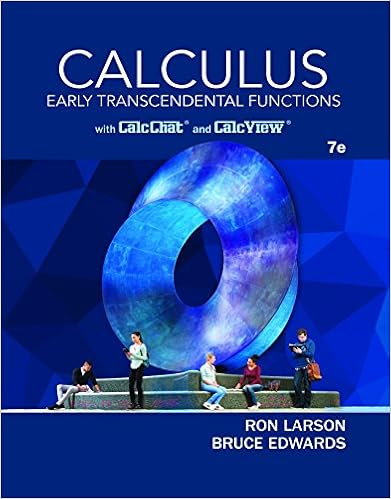# HW6_Sol.pdf - Winter 2018 EE 236A Christina Fragouli Linear...

• 10
• 96% (23) 22 out of 23 people found this document helpful

This preview shows page 1 - 3 out of 10 pages.

##### We have textbook solutions for you!
The document you are viewing contains questions related to this textbook.The document you are viewing contains questions related to this textbook.
Chapter 15 / Exercise 26
Calculus: Early Transcendental Functions
Edwards/LarsonExpert Verified
Winter 2018EE 236AChristina FragouliLinear ProgrammingSolutions of Homework 6Problem 1(4 points): As we discussed in class, the following LPmaximizefisubject toi:ePifice,edgesefi0(1)is the max-flow problem formulation with flows associated with paths, whereP={Pi}is the setof all paths from source to destination, andfiis the flow associated with theith path.(1) Prove that the LP in (1) is equivalent to the max-flow problem formulation with flows associatedto each edge (provided in Problem 1).(2) In a graph with unit capacity edges (i.e.,ce= 1,edgese), prove that if the the solutionof the max-flow problem iskthen we can findkedge-disjoint paths fromstotin the graph.(Two paths are said to be edge-disjoint if they do not share a common edge.)(3) Derive the dual of (1) and give an interpretation to the dual variables and the objective function.
##### We have textbook solutions for you!
The document you are viewing contains questions related to this textbook.The document you are viewing contains questions related to this textbook.
Chapter 15 / Exercise 26
Calculus: Early Transcendental Functions
Edwards/LarsonExpert Verified
Next, we show the equivalence.(1). P2P1: Assume an optimal solutionf*ijexists for the max-flow problem. Then constructthe set of path flowsfmfor allmin such a way that the following holdsXm: (i,j)Pmfm=f?ij,for all (i, j)∈ E.(4)If such a construction offmis possible, then we can proceed by proving the equivalence. It is easyto see that this selection offmfor allmis greater than or equal to 0, and satisfies the capacityconstraint in (1). Therefore, that construction gives a feasible point in (1). Note that each pathends at the destination, and therefore for eachPm, there existsisuch that (i, d)Pm. Therefore,the objective function can be expressed asXfm=Xi:(i,d)∈EXm:(i,d)Pmfm=Xi:(i,d)∈Ef?id=f?dswhere the second equality follows from (2) and last equality follows from (3). What remains is totry to prove that such a construction offmwith condition (4) is possible. One way to constructsuch a solution is using the following algorithm1. Initializeˆfij=f?ijfor all (i, j)∈ Eandfm= 0 for all pathsm.2. Select a pathPm.For pathPm, select the edge (i, j)minPmsuch that (i, j)min=argmin(i,j)Pmˆfij(the minimum edge flow across the path).Set the flow of the path to beequal to that edge flow,i.e.,fm=ˆf(i,j)min.3. Subtractˆf(i,j)minfromˆfijfor all (i, j)Pm(i.e., subtract it from all edge flows of all edgesin the path).4. Repeat the previous steps until in every path there is at least one edge withˆfij= 0.
•••